# Introduction to Deep Learning: Feed Forward Neural Networks FFNNs (a.k.a.

Source: Deep Learning on Medium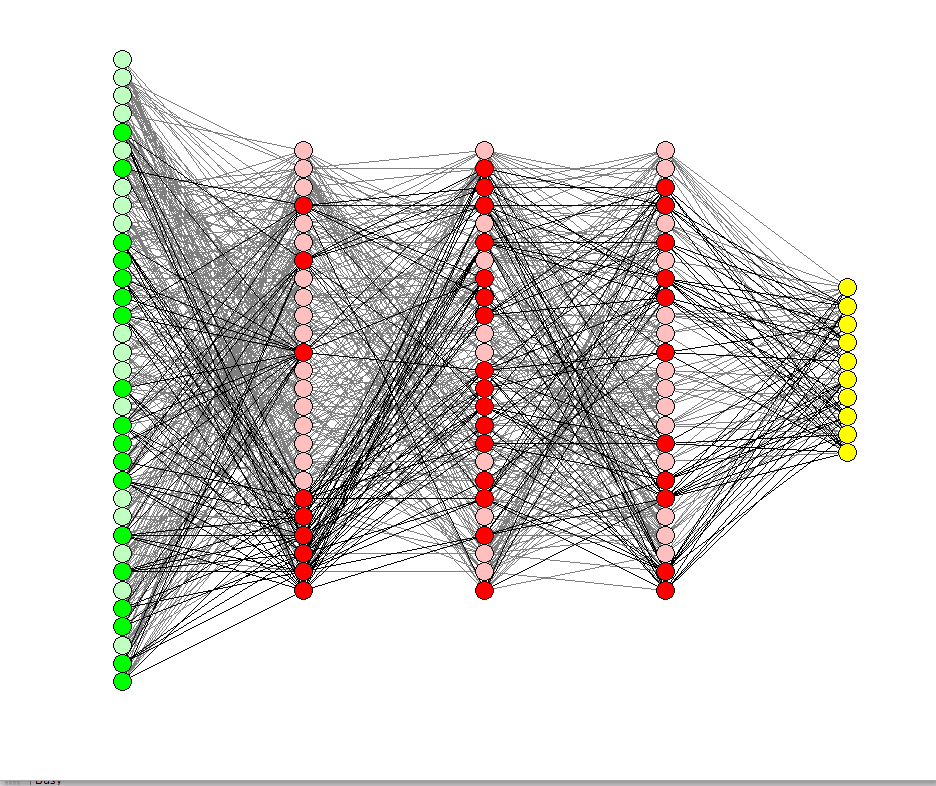A Deep Feed Forward Neural Network (FFNN) — aka Multi-Layered Perceptron (MLP)

An Artificial Neural Network (ANN) is made of many interconnected neurons: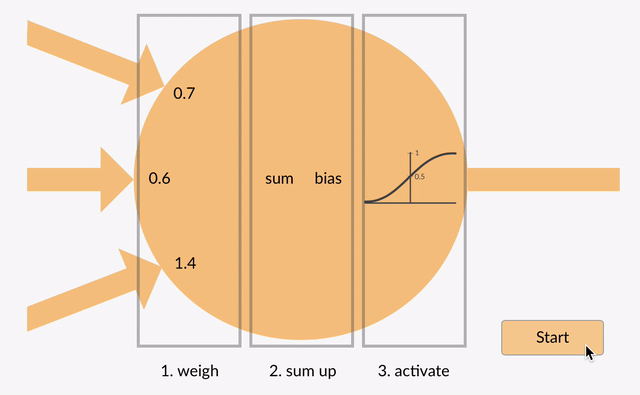A single Neuron from an Artificial Neural Network (ANN)

Each neuron takes in some floating point numbers (e.g. 1.0, 0.5, -1.0) and multiplies them by some other floating point numbers (e.g. 0.7, 0.6, 1.4) known as weights (1.0 * 0.7 = 0.7, 0.5 * 0.6 = 0.3, -1.0 * 1.4 = -1.4). The weights act as a mechanism to focus on, or ignore, certain inputs. The weighted inputs then get summed together (e.g. 0.7 + 0.3 + -1.4 = -0.4) along with a bias value (e.g. -0.4 + -0.1 = -0.5).

The summed value (x) is now transformed into an output value (y) according to the neuron’s activation function (y = f(x)). Some popular activation functions are shown below: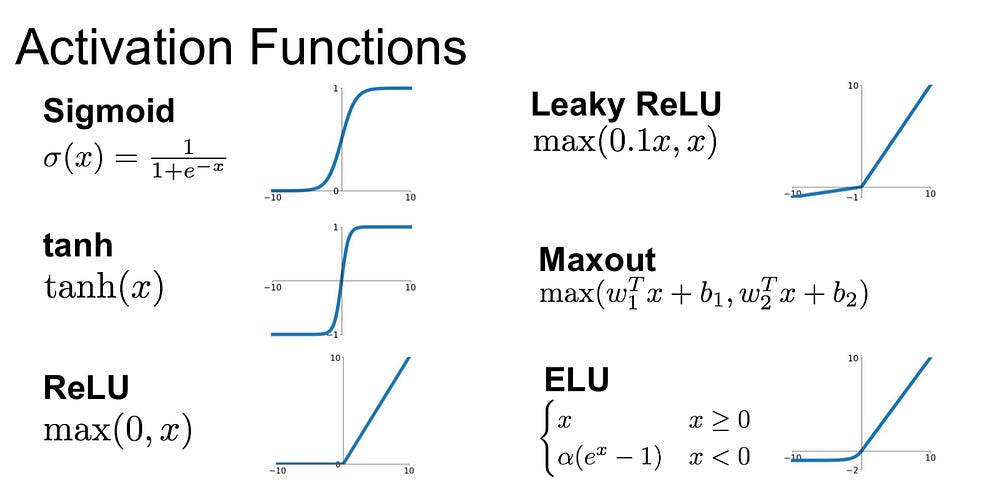A small selection of Popular Activation Functions

e.g. -0.5 → -0.05 if we use the Leaky Rectified Linear Unit (Leaky ReLU) activation function: y = f(x) = f(-0.5) = max(0.1*-0.5, -0.5) = max(-0.05, -0.5) = -0.05

The neuron’s output value (e.g. -0.05) is often an input for another neuron.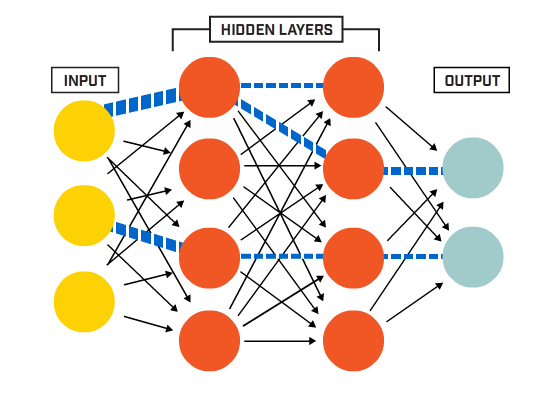A Neuron’s output value often feeds in as an input to other Neurons in the Artificial Neural Network (ANN)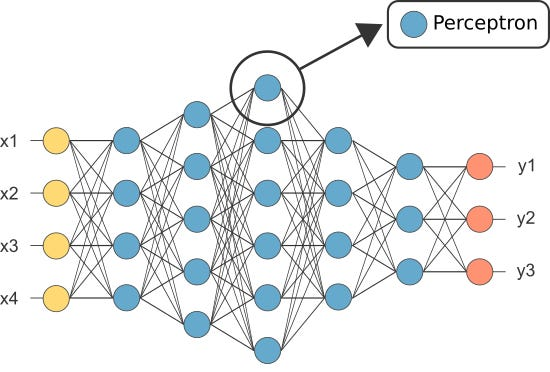The Perceptron, one of the first Neural Networks, is made of just a single Neuron

However, one of the first ANNs was known as the perceptron and it consisted of only a single neuron.

The output of the perceptron’s (only) neuron acts as the final prediction.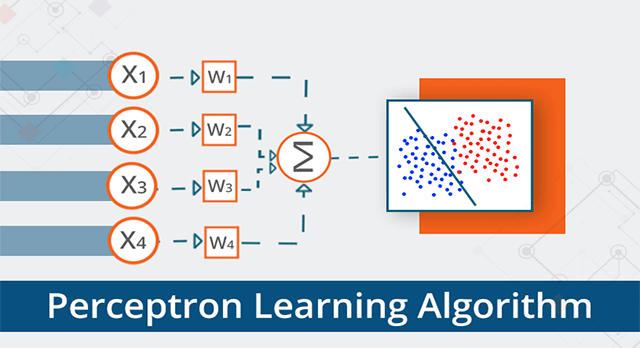Each Neuron is a linear binary classifier all on its own (e.g. an output value >= 0 indicates the blue class, while an output value < 0 indicates the red class)

Lets code our own Perceptron:

`import numpy as np`
`class Neuron:  def __init__(self, n_inputs, bias = 0., weights = None):  self.b = bias if weights: self.ws = np.array(weights) else: self.ws = np.random.rand(n_inputs) def __call__(self, xs):  return self._f(xs @ self.ws + self.b)  def _f(self, x):  return max(x*.1, x)`

(Note: we have not included any learning algorithm in our example above — we shall cover learning algorithms in another tutorial)

`perceptron = Neuron(n_inputs = 3, bias = -0.1, weights = [0.7, 0.6, 1.4])`
`perceptron([1.0, 0.5, -1.0])`

-0.04999999999999999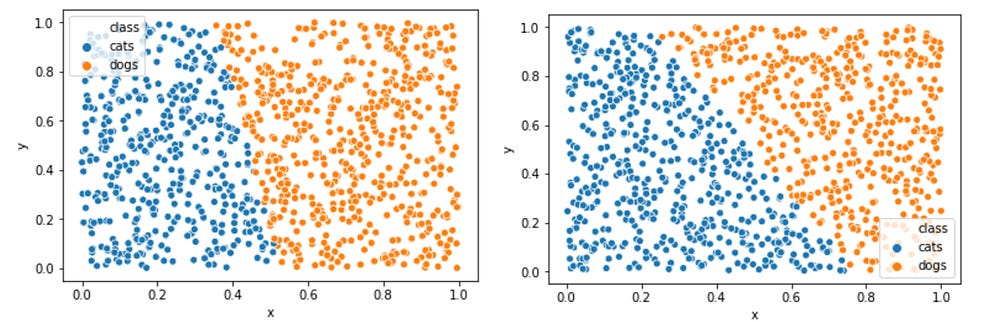Notice that by adjusting the values of the weights and bias, you can adjust the neuron’s decision boundary. (NB: a neuron learns by updating its weights and bias values to reduce the error of its decisions).

So why do we need so many neurons in an ANN if any one will suffice (as a classifier)?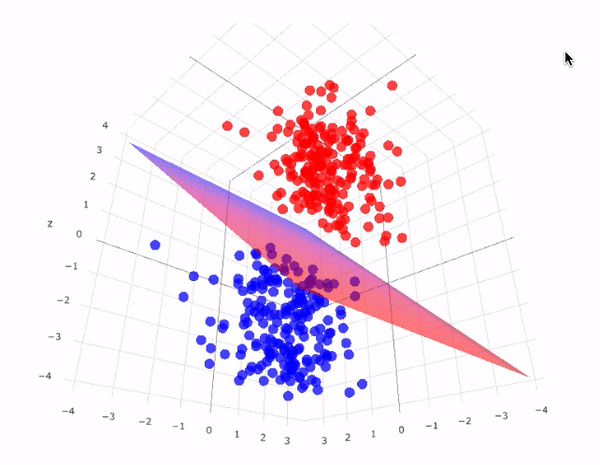Limitations: The neuron is a binary classifier since it can only learn to distinguish between two classes (e.g. blue and red) max. The neuron is a linear classifier because it’s decision boundary is a straight line for 2D data (or a flat plane for 3D data, etc)

Unfortunately, individual neurons are unable to classify non-linearly separable data because they can only ever learn a linear decision boundary.

However, by combining neurons together, we essentially combine their decision boundaries. Therefore, an ANN composed of many neurons is able to learn non-linear decision boundaries.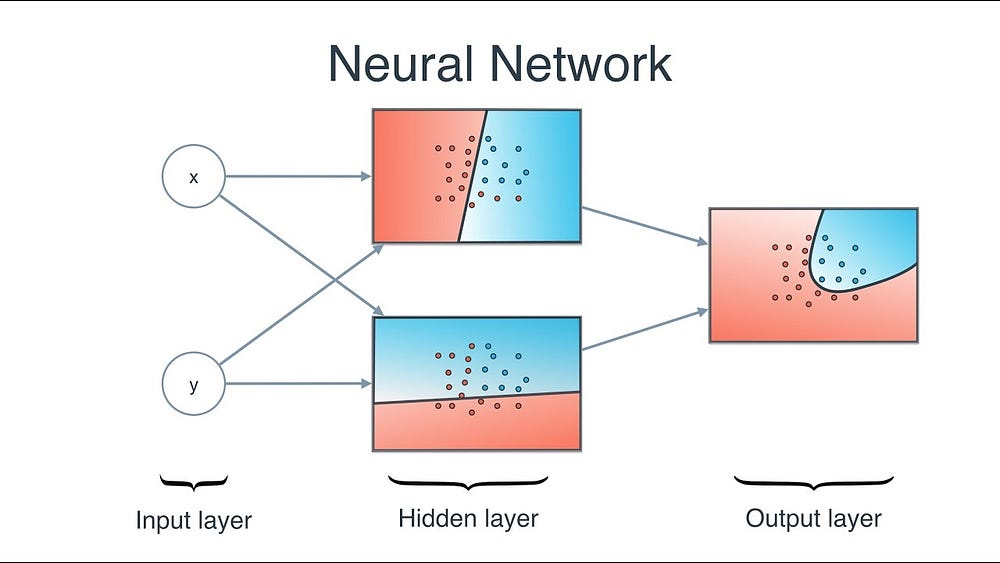Combining Neurons allows Neural Networks to learn Nonlinear Decision Boundaries

Neurons are connected together according to a specific network architecture. Though there are different architectures, nearly all of them contain layers. (NB: Neurons in the same layer do not connect with one another)

There is typically an input layer (containing a number of neurons equal to the number of input features in the data), an output layer (containing a number of neurons equal to the number of classes) and a hidden layer (containing any number of neurons).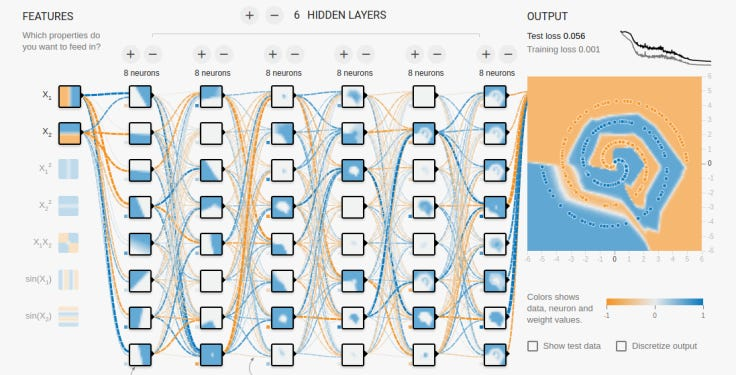Deep neural networks contain multiple hidden layers

There can be more than one hidden layer to allow the neural net to learn more complex decision boundaries (Any neural net with more than one hidden layer is considered a deep neural net).

Lets build a deep NN to paint this picture:(No we won’t use GANs to paint this — we will just use a deep Feed Forward Neural Net (FFNN) and treat this like a fancy classification problem!

Lets download the image and load its pixels into an array

`!curl -O https://pmcvariety.files.wordpress.com/2018/04/twitter-logo.jpg?w=100&h=100&crop=1`
`from PIL import Imageimage = Image.open('twitter-logo.jpg?w=100')`
`import numpy as npimage_array = np.asarray(image)`

Now teaching our ANN to paint is a supervised learning task, so we need to create a labelled training set (Our training data will have inputs and expected output labels for each input). The training inputs will have 2 values (the x,y coordinates of each pixel) and the training outputs will have 3 values (the r,g,b colour values for each pixel). Our ANN will essentially learn to associate certain colours to certain regions of the picture.

`training_inputs,training_outputs = [],[]for row,rgbs in enumerate(image_array): for column,rgb in enumerate(rgbs): training_inputs.append((row,column)) r,g,b = rgb training_outputs.append((r/255,g/255,b/255))`

Now lets create our ANN: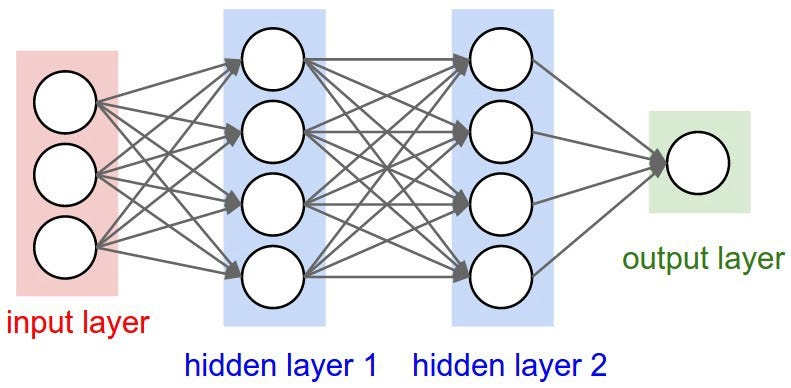A fully-connected feed-forward neural network (FFNN) — aka A multi-layered perceptron (MLP)
• It should have 2 neurons in the input layer (since there are 2 values to take in: x & y coordinates).
• It should have 3 neurons in the output layer (since there are 3 values to learn: r, g, b).
• The number of hidden layers and the number of neurons in each hidden layer are two hyperparameters to experiment with (as well as the number of epochs we will train it for, the activation function, etc) — I’ll use 10 hidden layers with 100 neurons in each hidden layer (making this a deep neural network)
`from sklearn.neural_network import MLPRegressor`
`ann = MLPRegressor(hidden_layer_sizes= tuple(100 for _ in range(10)))`
`ann.fit(training_inputs, training_outputs)`

The trained network can now predict the normalised rgb values for any coordinates (e.g. x,y = 1,1).

`ann.predict([[1,1]])`

array([[0.95479563, 0.95626562, 0.97069882]])

lets use the ANN to predict the rgb values for every coordinate and lets display the predicted rgb values for the entire image to see how well it (qualitatively)

`predicted_outputs = ann.predict(training_inputs)`
`predicted_image_array = np.zeros_like(image_array)i = 0for row,rgbs in enumerate(predicted_image_array): for column in range(len(rgbs)): r,g,b = predicted_outputs[i] predicted_image_array[row][column] = [r*255,g*255,b*255] i += 1Image.fromarray(predicted_image_array)`Our ANN’s Painting (predicted pixel colours)

Try changing the hyperparameters to get better results. Enjoy!

You can find all the code in this tutorial on a notebook which can be run via Google Colab here: https://colab.research.google.com/github/mohammedterry/ANNs/blob/master/ML_ANN.ipynb